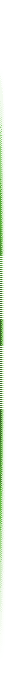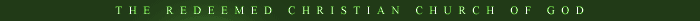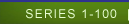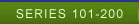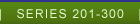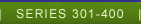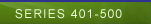LESSON 401-425 Select Lesson Lesson 401 Lesson 402 Lesson 403 Lesson 404 Lesson 405 Lesson 406 Lesson 407 Lesson 408 Lesson 409 Lesson 140 Lesson 411 Lesson 412 Lesson 413 Lesson 414 Lesson 415 Lesson 416 Lesson 417 Lesson 418 Lesson 419 Lesson 420 Lesson 421 Lesson 422 Lesson 423 Lesson 424 Lesson 425 LESSON 426- 450 Select Lesson Lesson 426 Lesson 427 Lesson 428 Lesson 429 Lesson 430 Lesson 431 Lesson 432 Lesson 433 Lesson 434 Lesson 435 Lesson 436 Lesson 437 Lesson 438 Lesson 439 Lesson 440 Lesson 441 Lesson 442 Lesson 443 Lesson 444 Lesson 445 Lesson 446 Lesson 447 Lesson 448 Lesson 449 Lesson 450 LESSON 451-475 Select Lesson Lesson 451 Lesson 452 Lesson 453 Lesson 454 Lesson 455 Lesson 456 Lesson 457 Lesson 458 Lesson 459 Lesson 460 Lesson 461 Lesson 462 Lesson 463 Lesson 464 Lesson 465 Lesson 466 Lesson 467 Lesson 468 Lesson 469 Lesson 470 Lesson 471 Lesson 472 Lesson 473 Lesson 474 Lesson 475 LESSON 476-500 Select Lesson Lesson 476 Lesson 477 Lesson 478 Lesson 479 Lesson 480 Lesson 481 Lesson 482 Lesson 483 Lesson 484 Lesson 485 Lesson 486 Lesson 487 Lesson 488 Lesson 489 Lesson 490 Lesson 491 Lesson 492 Lesson 493 Lesson 494 Lesson 495 Lesson 496 Lesson 497 Lesson 498 Lesson 499 Lesson 500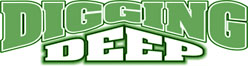LESSON 401 OIL - MARK OF THE HOLY SPIRIT INTRODUCTION: We are in the second part of the mark of the Holy Spirit, which is oil. In this study, we would talk about key elements of the oil of the spirit. May the Lord bless you as you study at His feet in Jesus Name, Amen. 1. KEY ELEMENTS OF OIL OF THE SPIRIT Oil is sometimes used for a setting apart, believers are meant to set themselves apart for the Lord. They are to be set apart for the work of the Lord, a wise believer will constantly have oil in his lamp to shine for the Lord. Ex.32;29, Rom.12:1, Judges 5:2, II Cor.8:5, II Tim. 2:21, II Cor.15:12-15, Is.10:27, Jam. 5:14-15, Is.61:1-3, Heb.1:9, Jam.5:14-16, Ps.45:7, Mt. 25:3-4, Dan.12:3, Mt.5:14-16, Mt. 13:43, Mt. 25:3, Ps. 23:6, Ex. 35:8